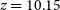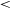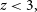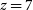Hostname: page-component-797576ffbb-cx6qr Total loading time: 0 Render date: 2023-12-02T22:06:22.417Z Has data issue: false Feature Flags: { "corePageComponentGetUserInfoFromSharedSession": true, "coreDisableEcommerce": false, "useRatesEcommerce": true } hasContentIssue false

# The nature and likely redshift of GLEAM J0917–0012

Published online by Cambridge University Press:  21 September 2021

*
*Author for correspondence: Guillaume Drouart, E-mail: guillaume.drouart@curtin.edu.au

## Abstract

We previously reported a putative detection of a radio galaxy at$z=10.15$ , selected from the GaLactic and Extragalactic All-sky Murchison Widefield Array (GLEAM) survey. The redshift of this source, GLEAM J0917–0012, was based on three weakly detected molecular emission lines observed with the Atacama Large Millimetre Array (ALMA). In order to confirm this result, we conducted deep spectroscopic follow-up observations with ALMA and the Karl Jansky Very Large Array (VLA). The ALMA observations targeted the same CO lines previously reported in Band 3 (84–115 GHz) and the VLA targeted the CO(4-3) and [CI(1-0)] lines for an independent confirmation in Q-band (41 and 44 GHz). Neither observation detected any emission lines, removing support for our original interpretation. Adding publicly available optical data from the Hyper Suprime-Cam survey, Widefield Infrared Survey Explorer (WISE), and Herschel Space Observatory in the infrared, as well as$<$ 10 GHz polarisation and 162 MHz inter-planetary scintillation observations, we model the physical and observational characteristics of GLEAM J0917–0012 as a function of redshift. Comparing these predictions and observational relations to the data, we are able to constrain its nature and distance. We argue that if GLEAM J0917–0012 is at$z<3,$ then it has an extremely unusual nature, and that the more likely solution is that the source lies above$z=7$ .

Type
Research Article
© The Author(s), 2021. Published by Cambridge University Press on behalf of the Astronomical Society of Australia

## Access options

Get access to the full version of this content by using one of the access options below. (Log in options will check for institutional or personal access. Content may require purchase if you do not have access.)

## References

Aihara, H., et al. 2018, PASJ, 70, S8 Google Scholar
Allen, R. J., et al. 2017, ApL, 834, L11 CrossRefGoogle Scholar
AAlvarez MAarquez, J., Burgarella, D., Buat, V., Ilbert, O., & Perez-GonzAalez, P. G. 2019, A&A, 630, A153CrossRefGoogle Scholar
Antonucci, R. 1993, ARA&A, 31, 473 CrossRefGoogle Scholar
Arnaboldi, M., Neeser, M. J., Parker, L. C., Rosati, P., Lombardi, M., Dietrich, J. P., & Hummel, W. 2007, The Messenger, 127, 28 Google Scholar
Becker, R. H., White, R. L., & Helfand, D. J. 1995, ApJ, 450, 559 CrossRefGoogle Scholar
Bianchi, S. 2013, A&A, 552, A89 CrossRefGoogle Scholar
Blain, A. W., Smail, I., Ivison, R. J., & Kneib, J.-P. 1999, MNRAS, 302, 632 CrossRefGoogle Scholar
Brammer, G. B., van Dokkum, P. G., & Coppi, P. 2008, ApJ, 686, 1503 CrossRefGoogle Scholar
Buat, V., et al., 2011, A&A, 533, A93 CrossRefGoogle Scholar
Cañameras, R., et al. 2021, A&A, 645, A45CrossRefGoogle Scholar
Cappelluti, N., et al. 2011, MSAIS, 17, 159 CrossRefGoogle Scholar
Carilli, C. L., & Walter, F. 2013, ARA&A, 51, 105 CrossRefGoogle Scholar
Chambers, K. C., et al. 2016, eprint arXiv:1612.05560, p. arXiv:1612.05560Google Scholar
Chhetri, R., Morgan, J., Ekers, R. D., Macquart, J.-P., Sadler, E. M., Giroletti, M., Callingham, J. R., & Tingay, S. J. 2018, MNRAS, 474, 4937 CrossRefGoogle Scholar
Condon, J. J., Cotton, W. D., Greisen, E. W., Yin, Q. F., Perley, R. A., Taylor, G. B., & Broderick, J. J., 1998, AJ, 115, 1693 CrossRefGoogle Scholar
da Cunha, E., et al. 2013, ApJ, 766, 13 CrossRefGoogle Scholar
De Breuck, C., van Breugel, W., Röttgering, H. J. A., & Miley, G. 2000, A&AS, 143, 303CrossRefGoogle Scholar
De Breuck, C., van Breugel, W., Stanford, S. A., Röttgering, H., Miley, G., & Stern, D. 2002, AJ, 123, 637CrossRefGoogle Scholar
De Breuck, C., et al. 2010, ApJ, 725, 36 CrossRefGoogle Scholar
Draine, B. T., & Li, A. 2007, ApJ, 657, 810 CrossRefGoogle Scholar
Driver, S. P., et al. 2016, MNRAS, 455, 3911 Google Scholar
Drouart, G., & Falkendal, T. 2018, Astrophysics Source Code Library, p. ascl:1809.015Google Scholar
Drouart, G., et al. 2012, A&A, 548, A45 CrossRefGoogle Scholar
Drouart, G., et al. 2014, A&A, 566, A53 CrossRefGoogle Scholar
Drouart, G., Rocca-Volmerange, B., De Breuck, C., Fioc, M., Lehnert, M., Seymour, N., Stern, D., & Vernet, J. 2016, A&A, 593, A109 CrossRefGoogle Scholar
Drouart, G., et al. 2020, PASA, 37, e026 Google Scholar
Eales, S. A. 1985, MNRAS, 217, 149 CrossRefGoogle Scholar
Eisenhardt, P. R. M., et al. 2012, ApJ, 755, 173 CrossRefGoogle Scholar
Elvis, M., et al. 1994, ApJS, 95, 1 CrossRefGoogle Scholar
Emonts, B. H. C., et al. 2014, MNRAS, 438, 2898 Google Scholar
Falkendal, T., et al. 2019, A&A, 621, A27 CrossRefGoogle Scholar
Fan, X., Carilli, C. L., & Keating, B. 2006, ARA&A, 44, 415 CrossRefGoogle Scholar
Fan, L., Han, Y., Nikutta, R., Drouart, G., & Knudsen, K. K. 2016, ApJ, 823, 107 CrossRefGoogle Scholar
Ferrara, A., Bianchi, S., Cimatti, A., & Giovanardi, C., 1999, ApJS, 123, 437 CrossRefGoogle Scholar
Fioc, M., & Rocca-Volmerange, B. 1997, A&A, 326, 950 Google Scholar
Fioc, M., & Rocca-Volmerange, B. 2019, A&A, 623, A143 CrossRefGoogle Scholar
Fitzpatrick, E. L. 1999, PASP, 111, 63 CrossRefGoogle Scholar
Franzen, T. M. O., et al. 2021, arXiv e-prints, p. arXiv:2107.09030 Google Scholar
Gordon, Y. A., et al. 2020, RNAAS, 4, 175 Google Scholar
Gullberg, B., et al. 2016, A&A, Volume 591, id.A73, 13 pp., 591, A73CrossRefGoogle Scholar
Hancock, P. J., Trott, C. M., & Hurley-Walker, N. 2018, PASA, 35, e011 CrossRefGoogle Scholar
Hurley-Walker, N., et al. 2017, MNRAS, 464, 1146 Google Scholar
Ighina, L., Belladitta, S., Caccianiga, A., Broderick, J. W., Drouart, G., Moretti, A., & Seymour, N. 2021, arXiv e-prints, 2101, arXiv:2101.11371Google Scholar
Intema, H. T., Jagannathan, P., Mooley, K. P., & Frail, D. A. 2017, A&A, 598, A78 CrossRefGoogle Scholar
Kaiser, C. R., & Best, P. N. 2007, MNRAS, 381, 1548 CrossRefGoogle Scholar
Krips, M., Neri, R., & Cox, P. 2012, ApJ, 753, 135 CrossRefGoogle Scholar
Lehnert, M. D., Yang, C., Emonts, B. H. C., Omont, A., Falgarone, E., Cox, P., & Guillard, P. 2020, A&A, 641, A124 CrossRefGoogle Scholar
Lilly, S. J., & Longair, M. S. 1984, MNRAS, 211, 833 CrossRefGoogle Scholar
Manchester, R. N., Hobbs, G. B., Teoh, A., & Hobbs, M. 2005, AJ, 129, 1993 CrossRefGoogle Scholar
Mauch, T., Klöckner, H.-R., Rawlings, S., Jarvis, M., Hardcastle, M. J., Obreschkow, D., Saikia, D. J., & Thompson, M. A. 2013, MNRAS, 435, 650 CrossRefGoogle Scholar
Mazzarella, J. M., Graham, J. R., Sanders, D. B., & Djorgovski, S. 1993, ApJ, 409, 170 CrossRefGoogle Scholar
McConnell, D., et al. 2020, PASA, 37, e048 CrossRefGoogle Scholar
McMullin, J. P., Waters, B., Schiebel, D., Young, W., & Golap, K. 2007, Astron. Data Anal. Softw. Syst XVI, 376, 127Google Scholar
Meyer, M., Robotham, A., Obreschkow, D., Westmeier, T., Duffy, A. R., & Staveley-Smith, L. 2017, PASA, 34CrossRefGoogle Scholar
Mezcua, M., Suh, H., Civano, F. 2019, MNRAS, 488, 685 CrossRefGoogle Scholar
Mignani, R. P., De Luca, A., Hummel, W., Zajczyk, A., Rudak, B., Kanbach, G., & Slowikowska, A. 2012, A&A, 544, A100 CrossRefGoogle Scholar
Miley, G., & De Breuck, C. 2008, A&ARv, 15, 67 Google Scholar
Morgan, J. S., et al. 2018, MNRAS, 473, 2965 Google Scholar
Murphy, T., et al. 2017, PASA, 34, e020 Google Scholar
Nesvadba, N. P. H., De Breuck, C., Lehnert, M. D., Best, P. N., Binette, L., & Proga, D. 2011, A&A, 525, A43 CrossRefGoogle Scholar
Pilbratt, G. L., et al. 2010, A&A, 518, L1 CrossRefGoogle Scholar
Planck, Collaboration et al. 2016, A&A, 594, A13 Google Scholar
Pâris, I., et al. 2018, A&A, 613, A51Google Scholar
Purcell, C. R., Van Eck, C. L., West, J., Sun, X. H., & Gaensler, B. M. 2020, Astrophys. Source Code Library, p. ascl:2005.003Google Scholar
Riechers, D. A., Weib, A., Walter, F., & Wagg, J. 2010, ApJ, 725, 1032CrossRefGoogle Scholar
Riseley, C. J., et al. 2020, PASA, 37, e029 Google Scholar
Rocca-Volmerange, B., Le Borgne, D., De Breuck, C., Fioc, M., & Moy, E. 2004, A&A, 415, 931 CrossRefGoogle Scholar
Saxena, A., Röttgering, H. J. A., & Rigby, E. E. 2017, MNRAS, 469, 4083CrossRefGoogle Scholar
Saxena, A., et al., 2018a, MNRAS, 475, 5041 Google Scholar
Saxena, A., et al. 2018b, MNRAS, 480, 2733 Google Scholar
Saxena, A., et al. 2019, arXiv e-prints, p. arXiv:1906.00746Google Scholar
Seymour, N., et al. 2007, ApJS, 171, 353 Google Scholar
Shimwell, T. W., et al. 2017, A&A, 598, A104 Google Scholar
Shimwell, T. W., et al. 2019, A&A, 622, A1 Google Scholar
Skrutskie, M. F., et al. 2006, AJ, 131, 1163 Google Scholar
Solomon, P. M., & Vanden Bout, P. A. 2005, ARA&A, 43, 677 CrossRefGoogle Scholar
Spilker, J. S., et al. 2014, ApJ, 785, 149 Google Scholar
Spingola, C., Dallacasa, D., Belladitta, S., Caccianiga, A., Giroletti, M., Moretti, A., & Orienti, M. 2020, A&A, 643, L12 CrossRefGoogle Scholar
Stern, D., Walton, D. J. 2020, ApL, 895, L38 CrossRefGoogle Scholar
Stern, D., et al. 2005, ApJ, 631, 163 CrossRefGoogle Scholar
Tingay, S. J., et al. 2013, PASA, 30, e007 Google Scholar
Tsai, C.-W., et al. 2015, ApJ, 805, 90 CrossRefGoogle Scholar
Volonteri, M., & Rees, M. J. 2005, ApJ, 633, 624 CrossRefGoogle Scholar
Wang, S. X., et al. 2013, ApJ, 778, 179 CrossRefGoogle Scholar
Wang, F., et al. 2021, ApL, 907, L1Google Scholar
Wayth, R. B., et al. 2015, PASA, 32, e025 Google Scholar
Weib, A., et al. 2013, ApJ, 767, 88Google Scholar
Wright, E. L., et al. 2010, AJ, 140, 1868 Google Scholar
Wylezalek, D., et al. 2014, ApJ, 786, 17 CrossRefGoogle Scholar
Yang, C., Gao, Y., Omont, A., Liu, D., Isaak, K. G., Downes, D., van der Werf, P. P., & Lu, N. 2013, ApL, 771, L24 CrossRefGoogle Scholar
van Breugel, W., De Breuck, C., Stanford, S. A., Stern, D., Röttgering, H., Miley, G. 1999, ApJ, 518, L61CrossRefGoogle Scholar
van der Werf, P. P., et al. 2011, ApL, 741, L38 CrossRefGoogle Scholar
Zhang, Z.-Y., Papadopoulos, P. P., Ivison, R. J., Galametz, M., Smith, M. W. L., Xilouris, E. M. 2016, Royal Society Open Science, 3, 160025 CrossRefGoogle Scholar